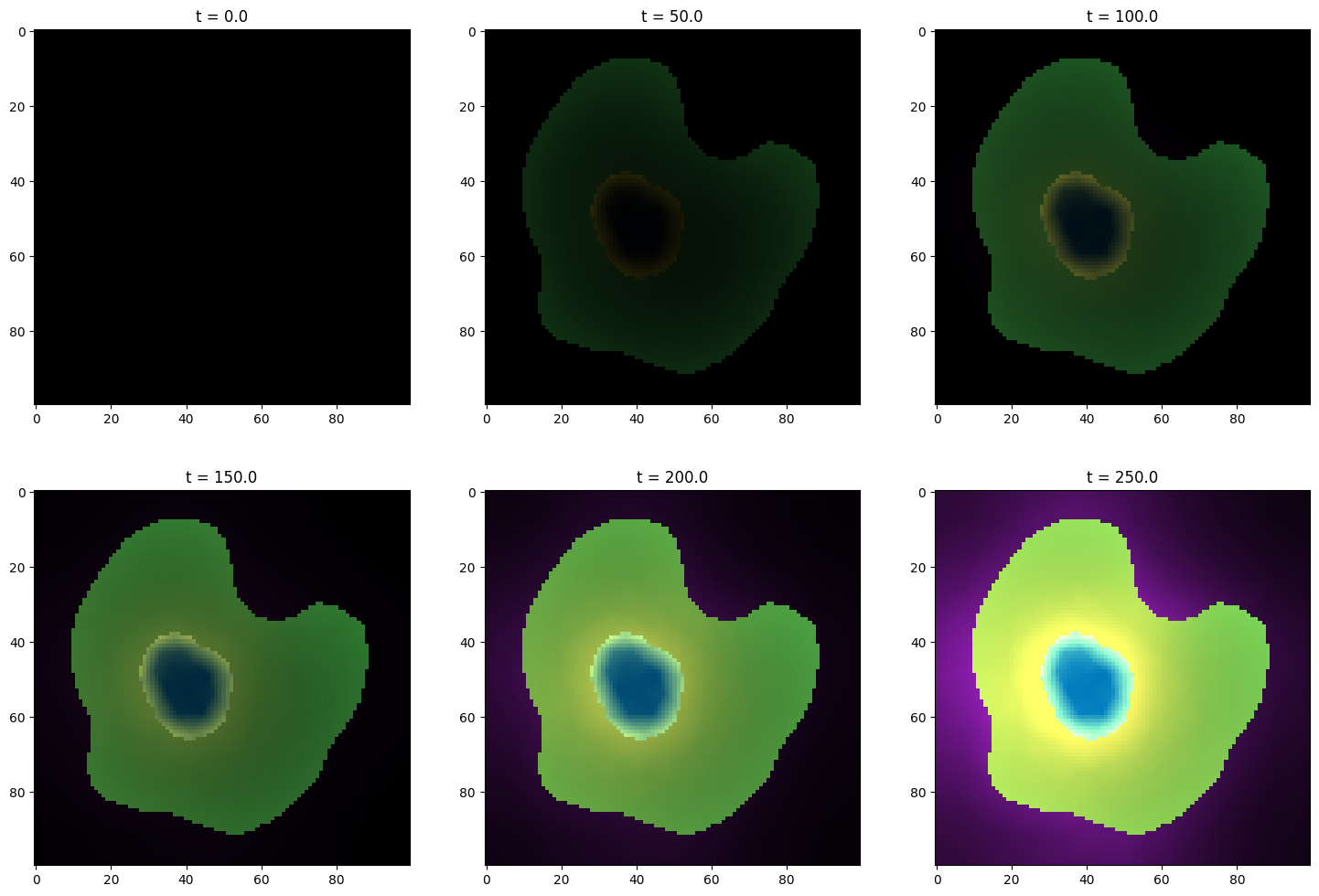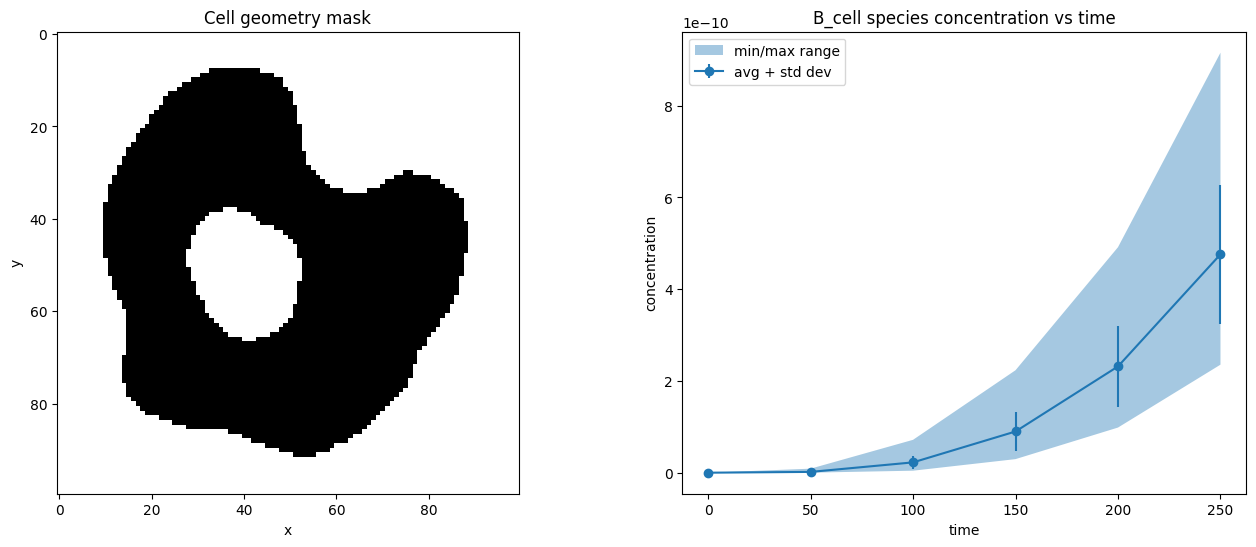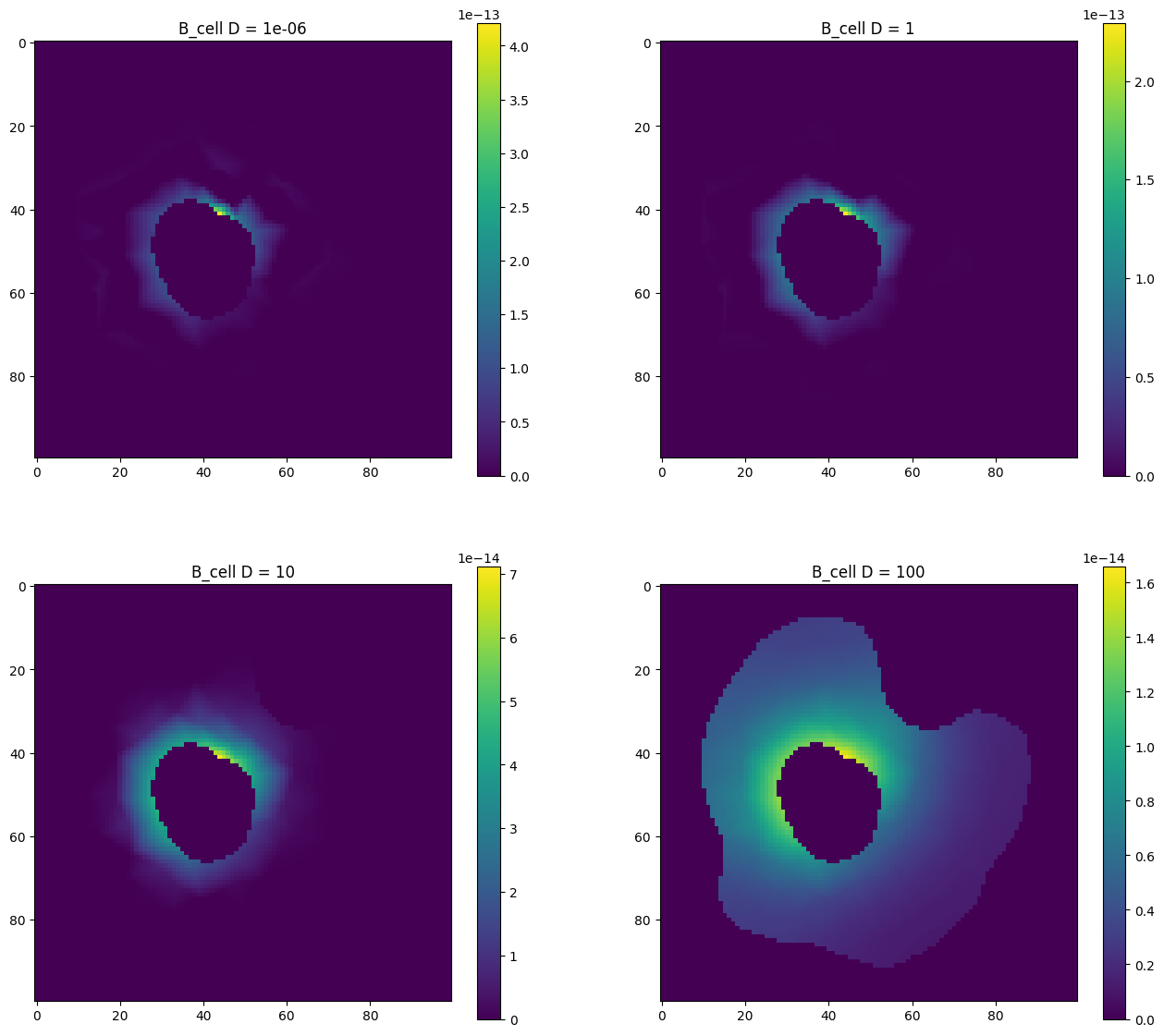# Simulating¶

## Open an example model¶

:

!pip install -q sme
import sme
from matplotlib import pyplot as plt
import numpy as np

:

my_model = sme.open_example_model()


## Running a simulation¶

• models can be simulated by specifying the total simulation time, and the interval between images

• the simulation returns a list of SimulationResult objects, each of which contains

• time_point: the time point

• concentration_image: an image of the species concentrations at this time point

• species_concentration: a dict of the concentrations for each species at this time point

:

sim_results = my_model.simulate(simulation_time=250.0, image_interval=50.0)
type(sim_results)
print(sim_results)

<sme.SimulationResult>
- timepoint: 0
- number of species: 5



### Display images from simulation results¶

:

fig, axs = plt.subplots(nrows=2, ncols=len(sim_results)//2, figsize=(18, 12))
for (ax, res) in zip(fig.axes, sim_results):
ax.imshow(res.concentration_image)
ax.set_title(f"t = {res.time_point}")
plt.show()### Plot concentrations from simulation results¶

:

result = sim_results
fig, axs = plt.subplots(nrows=3, ncols=2, figsize=(16, 20))
fig.delaxes(axs[2,1])
for (ax, (species, concentration)) in zip(fig.axes, result.species_concentration.items()):
im = ax.imshow(concentration)
ax.set_title(f"'{species}' at t = {result.time_point}")
fig.colorbar(im, ax=ax)
plt.show()### Plot average/min/max species concentrations¶

To get the average (or minimum/maxiumum/etc) concentration of a species in a compartment, we first use the compartment geometry_mask to only include the pixels that lie inside the compartment.

:

fig, (ax0, ax1) = plt.subplots(nrows=1, ncols=2, figsize=(16, 6))

# get mask of compartment pixels
ax0.set_xlabel("x")
ax0.set_ylabel("y")

# apply mask to results to get a flat array of all concentrations
# inside the compartment at each time point
times = []
concs = []
for result in sim_results:
times.append(result.time_point)

# calculate avg, min, max and plot
avg_conc = [np.mean(x) for x in concs]
std_conc = [np.std(x) for x in concs]
min_conc = [np.min(x) for x in concs]
max_conc = [np.max(x) for x in concs]
ax1.set_title("B_cell species concentration vs time")
ax1.set_xlabel("time")
ax1.set_ylabel("concentration")
ax1.errorbar(times, avg_conc, std_conc, label="avg + std dev", marker='o')
ax1.fill_between(times, min_conc, max_conc, label="min/max range", alpha=0.4)
ax1.legend()

plt.show()## Diffusion constant example¶

Here we repeat a simulation four times, each time with a different value for the diffusion constant of species B_cell, and plot the resulting concentration of this species at t=15.

:

diffconsts = [1e-6, 1, 10, 100]
fig, axs = plt.subplots(nrows=2, ncols=2, figsize=(16, 14))
for ax, diffconst in zip(fig.axes, diffconsts):
m = sme.open_example_model()
m.compartments['Cell'].species['B_cell'].diffusion_constant = diffconst
results = m.simulate(simulation_time=15.0, image_interval=15.0)
im = ax.imshow(results.species_concentration['B_cell'])
ax.set_title(f"B_cell D = {diffconst}")
fig.colorbar(im, ax=ax)
plt.show()